## Solving Systems of Equations (Simultaneous Equations)

If you have two different equations with the same two unknowns in each, you can solve for both unknowns. There are three common methods for solving: addition/subtraction, substitution, and graphing.

This method is also known as the elimination method.

To use the addition/subtraction method, do the following:

1. Multiply one or both equations by some number(s) to make the number in front of one of the letters (unknowns) the same or exactly the opposite in each equation.

2. Add or subtract the two equations to eliminate one letter.

3. Solve for the remaining unknown.

4. Solve for the other unknown by inserting the value of the unknown found in one of the original equations.

##### Example 1

Solve for x and y.Adding the equations eliminates the y‐terms.Now inserting 5 for x in the first equation gives the following:Answer: x = 5, y = 2

By replacing each x with a 5 and each y with a 2 in the original equations, you can see that each equation will be made true.

In Example and Example , a unique answer existed for x and y that made each sentence true at the same time. In some situations you do not get unique answers or you get no answers. You need to be aware of these when you use the addition/subtraction method.

##### Example 2

Solve for x and y.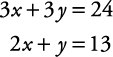First multiply the bottom equation by 3. Now the y is preceded by a 3 in each equation.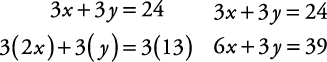The equations can be subtracted, eliminating the y terms.Insert x = 5 in one of the original equations to solve for y.Answer: x = 5, y = 3

Of course, if the number in front of a letter is already the same in each equation, you do not have to change either equation. Simply add or subtract.

To check the solution, replace each x in each equation with 5 and replace each y in each equation with 3.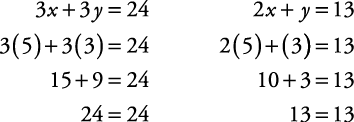##### Example 3

Solve for a and b.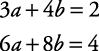Multiply the top equation by 2. Notice what happens.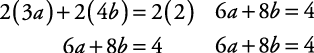Now if you were to subtract one equation from the other, the result is 0 = 0.

This statement is always true.

When this occurs, the system of equations does not have a unique solution. In fact, any a and b replacement that makes one of the equations true, also makes the other equation true. For example, if a = –6 and b = 5, then both equations are made true.

[3(– 6) + 4(5) = 2 AND 6(– 6) + 8(5) = 4]

What we have here is really only one equation written in two different ways. In this case, the second equation is actually the first equation multiplied by 2. The solution for this situation is either of the original equations or a simplified form of either equation.

##### Example 4

Solve for x and y.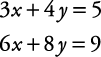Multiply the top equation by 2. Notice what happens.Now if you were to subtract the bottom equation from the top equation, the result is 0 = 1. This statement is never true. When this occurs, the system of equations has no solution.

In Examples 1–4, only one equation was multiplied by a number to get the numbers in front of a letter to be the same or opposite. Sometimes each equation must be multiplied by different numbers to get the numbers in front of a letter to be the same or opposite.

Solve for x and y.Notice that there is no simple number to multiply either equation with to get the numbers in front of x or y to become the same or opposites. In this case, do the following:

1. Select a letter to eliminate.

2. Use the two numbers to the left of this letter. Find the least common multiple of this value as the desired number to be in front of each letter.

3. Determine what value each equation needs to be multiplied by to obtain this value and multiply the equation by that number.

Suppose you want to eliminate x. The least common multiple of 3 and 5, the number in front of the x, is 15. The first equation must be multiplied by 5 in order to get 15 in front of x. The second equation must be multiplied by 3 in order to get 15 in front of x.Now subtract the second equation from the first equation to get the following:At this point, you can either replace y with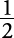and solve for x (method 1 that follows), or start with the original two equations and eliminate y in order to solve for x (method 2 that follows).

##### Method 1

Using the top equation: Replace y with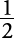and solve for x.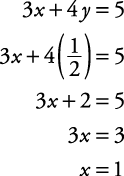##### Method 2

Eliminate y and solve for x.

The least common multiple of 4 and 6 is 12. Multiply the top equation by 3 and the bottom equation by 2.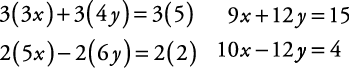Now add the two equations to eliminate y.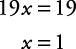The solution is x = 1 and.

#### Substitution method

Sometimes a system is more easily solved by the substitution method. This method involves substituting one equation into another.

##### Example 6

Solve for x and y.From the first equation, substitute ( y + 8) for x in the second equation.

( y + 8) + 3 y = 48

Now solve for y. Simplify by combining y's.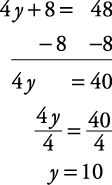Now insert y's value, 10, in one of the original equations.Answer: y = 10, x = 18

Check the solution.##### Example 7

Solve for x and y using the substitution method.First find an equation that has either a “1” or “ – 1” in front of a letter. Solve for that letter in terms of the other letter.

Then proceed as in example 6.

In this example, the bottom equation has a “1” in front of the y.

Solve for y in terms of x.Substitute 4 x – 17 for y in the top equation and then solve for x.Replace x with 4 in the equation y – 4 x = –17 and solve for y.The solution is x = 4, y = –1.

Check the solution:#### Graphing method

Another method of solving equations is by graphing each equation on a coordinate graph. The coordinates of the intersection will be the solution to the system. If you are unfamiliar with coordinate graphing, carefully review the articles on coordinate geometry before attempting this method.

##### Example 8

Solve the system by graphing.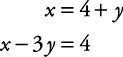First, find three values for x and y that satisfy each equation. (Although only two points are necessary to determine a straight line, finding a third point is a good way of checking.) Following are tables of x and y values:

x

y

4

0

2

–2

5

1

x

y

1

-1

4

0

7

1

Now graph the two lines on the coordinate plane, as shown in Figure 1.

The point where the two lines cross (4, 0) is the solution of the system.

If the lines are parallel, they do not intersect, and therefore, there is no solution to that system.